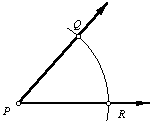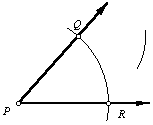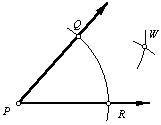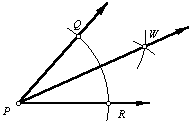Construct the bisector of an angle.

 1. Let point P be the vertex of the angle. Place the compass on point P and draw an arc across both sides of the angle. Label the intersection points Q and R.2. Place the compass on point Q and draw an arc across the interior of the angle.3. Without changing the radius of the compass, place it on point R and draw an arc intersecting the one drawn in the previous step. Label the intersection point W.4. Using the straightedge, draw ray PW. This is the bisector of ÐQPR.Back to Geometry Construction Reference